# Search by Topic

#### Resources tagged with 2D shapes and their properties similar to Equal Lengths:

Filter by: Content type:
Age range:
Challenge level:

### There are 67 results

Broad Topics > Angles, Polygons, and Geometrical Proof > 2D shapes and their properties### Floored

##### Age 11 to 14 Challenge Level:

A floor is covered by a tessellation of equilateral triangles, each having three equal arcs inside it. What proportion of the area of the tessellation is shaded?### Hex

##### Age 11 to 14 Challenge Level:

Explain how the thirteen pieces making up the regular hexagon shown in the diagram can be re-assembled to form three smaller regular hexagons congruent to each other.### Circle Packing

##### Age 14 to 16 Challenge Level:

Equal circles can be arranged so that each circle touches four or six others. What percentage of the plane is covered by circles in each packing pattern? ...### Square Pegs

##### Age 11 to 14 Challenge Level:

Which is a better fit, a square peg in a round hole or a round peg in a square hole?### Fitting In

##### Age 14 to 16 Challenge Level:

The largest square which fits into a circle is ABCD and EFGH is a square with G and H on the line CD and E and F on the circumference of the circle. Show that AB = 5EF. Similarly the largest. . . .### Circles, Circles Everywhere

##### Age 7 to 14

This article for pupils gives some examples of how circles have featured in people's lives for centuries.### Not So Little X

##### Age 11 to 14 Challenge Level:

Two circles are enclosed by a rectangle 12 units by x units. The distance between the centres of the two circles is x/3 units. How big is x?### Crescents and Triangles

##### Age 14 to 16 Challenge Level:

Can you find a relationship between the area of the crescents and the area of the triangle?### Arclets Explained

##### Age 11 to 16

This article gives an wonderful insight into students working on the Arclets problem that first appeared in the Sept 2002 edition of the NRICH website.### Semi-detached

##### Age 14 to 16 Challenge Level:

A square of area 40 square cms is inscribed in a semicircle. Find the area of the square that could be inscribed in a circle of the same radius.### Pent

##### Age 14 to 18 Challenge Level:

The diagram shows a regular pentagon with sides of unit length. Find all the angles in the diagram. Prove that the quadrilateral shown in red is a rhombus.### Rolling Around

##### Age 11 to 14 Challenge Level:

A circle rolls around the outside edge of a square so that its circumference always touches the edge of the square. Can you describe the locus of the centre of the circle?### Darts and Kites

##### Age 14 to 16 Challenge Level:

Explore the geometry of these dart and kite shapes!### Efficient Packing

##### Age 14 to 16 Challenge Level:

How efficiently can you pack together disks?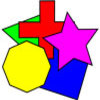### What Shape?

##### Age 7 to 14 Challenge Level:

This task develops spatial reasoning skills. By framing and asking questions a member of the team has to find out what mathematical object they have chosen.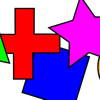### What Shape for Two

##### Age 7 to 14 Challenge Level:

'What Shape?' activity for adult and child. Can you ask good questions so you can work out which shape your partner has chosen?### Like a Circle in a Spiral

##### Age 7 to 16 Challenge Level:

A cheap and simple toy with lots of mathematics. Can you interpret the images that are produced? Can you predict the pattern that will be produced using different wheels?### Bow Tie

##### Age 11 to 14 Challenge Level:

Show how this pentagonal tile can be used to tile the plane and describe the transformations which map this pentagon to its images in the tiling.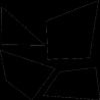### From One Shape to Another

##### Age 7 to 14

Read about David Hilbert who proved that any polygon could be cut up into a certain number of pieces that could be put back together to form any other polygon of equal area.### Tricircle

##### Age 14 to 16 Challenge Level:

The centre of the larger circle is at the midpoint of one side of an equilateral triangle and the circle touches the other two sides of the triangle. A smaller circle touches the larger circle and. . . .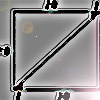### Lying and Cheating

##### Age 11 to 14 Challenge Level:

Follow the instructions and you can take a rectangle, cut it into 4 pieces, discard two small triangles, put together the remaining two pieces and end up with a rectangle the same size. Try it!### Squaring the Circle

##### Age 11 to 14 Challenge Level:

Bluey-green, white and transparent squares with a few odd bits of shapes around the perimeter. But, how many squares are there of each type in the complete circle? Study the picture and make. . . .### Some(?) of the Parts

##### Age 14 to 16 Challenge Level:

A circle touches the lines OA, OB and AB where OA and OB are perpendicular. Show that the diameter of the circle is equal to the perimeter of the triangle##### Age 14 to 16 Challenge Level:

Given a square ABCD of sides 10 cm, and using the corners as centres, construct four quadrants with radius 10 cm each inside the square. The four arcs intersect at P, Q, R and S. Find the. . . .### Square Areas

##### Age 11 to 14 Challenge Level:

Can you work out the area of the inner square and give an explanation of how you did it?### Tied Up

##### Age 14 to 16 Short Challenge Level:

How much of the field can the animals graze?### Circumspection

##### Age 14 to 16 Challenge Level:

M is any point on the line AB. Squares of side length AM and MB are constructed and their circumcircles intersect at P (and M). Prove that the lines AD and BE produced pass through P.### 2001 Spatial Oddity

##### Age 11 to 14 Challenge Level:

With one cut a piece of card 16 cm by 9 cm can be made into two pieces which can be rearranged to form a square 12 cm by 12 cm. Explain how this can be done.### Dividing the Field

##### Age 14 to 16 Challenge Level:

A farmer has a field which is the shape of a trapezium as illustrated below. To increase his profits he wishes to grow two different crops. To do this he would like to divide the field into two. . . .### Curvy Areas

##### Age 14 to 16 Challenge Level:

Have a go at creating these images based on circles. What do you notice about the areas of the different sections?### Rolling Coins

##### Age 14 to 16 Challenge Level:

A blue coin rolls round two yellow coins which touch. The coins are the same size. How many revolutions does the blue coin make when it rolls all the way round the yellow coins? Investigate for a. . . .##### Age 14 to 16 Challenge Level:

Investigate the properties of quadrilaterals which can be drawn with a circle just touching each side and another circle just touching each vertex.### Trapezium Four

##### Age 14 to 16 Challenge Level:

The diagonals of a trapezium divide it into four parts. Can you create a trapezium where three of those parts are equal in area?### From All Corners

##### Age 14 to 16 Challenge Level:

Straight lines are drawn from each corner of a square to the mid points of the opposite sides. Express the area of the octagon that is formed at the centre as a fraction of the area of the square.### LOGO Challenge - Circles as Animals

##### Age 11 to 16 Challenge Level:

See if you can anticipate successive 'generations' of the two animals shown here.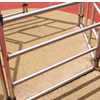### Playground Snapshot

##### Age 7 to 14 Challenge Level:

The image in this problem is part of a piece of equipment found in the playground of a school. How would you describe it to someone over the phone?### Rhombus in Rectangle

##### Age 14 to 16 Challenge Level:

Take any rectangle ABCD such that AB > BC. The point P is on AB and Q is on CD. Show that there is exactly one position of P and Q such that APCQ is a rhombus.### Semi-square

##### Age 14 to 16 Challenge Level:

What is the ratio of the area of a square inscribed in a semicircle to the area of the square inscribed in the entire circle?### Opposite Vertices

##### Age 11 to 14 Challenge Level:

Can you recreate squares and rhombuses if you are only given a side or a diagonal?##### Age 14 to 16 Challenge Level:

The sides of a triangle are 25, 39 and 40 units of length. Find the diameter of the circumscribed circle.### Squaring the Circle and Circling the Square

##### Age 14 to 16 Challenge Level:

If you continue the pattern, can you predict what each of the following areas will be? Try to explain your prediction.### Polycircles

##### Age 14 to 16 Challenge Level:

Show that for any triangle it is always possible to construct 3 touching circles with centres at the vertices. Is it possible to construct touching circles centred at the vertices of any polygon?### LOGO Challenge 8 - Rhombi

##### Age 7 to 16 Challenge Level:

Explore patterns based on a rhombus. How can you enlarge the pattern - or explode it?### Three Four Five

##### Age 14 to 16 Challenge Level:

Two semi-circles (each of radius 1/2) touch each other, and a semi-circle of radius 1 touches both of them. Find the radius of the circle which touches all three semi-circles.### The Medieval Octagon

##### Age 14 to 16 Challenge Level:

Medieval stonemasons used a method to construct octagons using ruler and compasses... Is the octagon regular? Proof please.### LOGO Challenge 2 - Diamonds Are Forever

##### Age 7 to 16 Challenge Level:

The challenge is to produce elegant solutions. Elegance here implies simplicity. The focus is on rhombi, in particular those formed by jointing two equilateral triangles along an edge.### Blue and White

##### Age 11 to 14 Challenge Level:

Identical squares of side one unit contain some circles shaded blue. In which of the four examples is the shaded area greatest?### Towering Trapeziums

##### Age 14 to 16 Challenge Level:

Can you find the areas of the trapezia in this sequence?### A Rational Search

##### Age 14 to 18 Challenge Level:

Investigate constructible images which contain rational areas.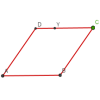### Roaming Rhombus

##### Age 14 to 16 Challenge Level:

We have four rods of equal lengths hinged at their endpoints to form a rhombus ABCD. Keeping AB fixed we allow CD to take all possible positions in the plane. What is the locus (or path) of the point. . . .